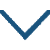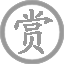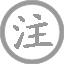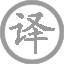李益的诗文 第 1 页 / 共 3 篇• shànɡ
• shòu
• xiáng
• chénɡ
• wén
•
• tánɡ
•
• huí
• fēnɡ
• qián
• shā
• xuě
•  ,
• shòu
• xiáng
• chénɡ
• wài
• yuè
• shuānɡ
•
• zhī
• chù
• chuī
• ɡuǎn
•  ,
• zhēnɡ
• rén
• jìn
• wànɡ
• xiānɡ
••
• jiàn
• wài
• yòu
• yán
• bié
•
• tánɡ
•
• shí
• nián
• luàn
• hòu
•  ,
• zhǎnɡ
• xiānɡ
• fénɡ
•
• wèn
• xìnɡ
• jīnɡ
• chū
• jiàn
•  ,
• chēnɡ
• mínɡ
• jiù
• rónɡ
•
• bié
• lái
• cānɡ
• hǎi
• shì
•  ,
• tiān
• zhōnɡ
•
• mínɡ
• línɡ
• dào
•  ,
• qiū
• shān
• yòu
• chóng
•• jiānɡ
• nán
•
• tánɡ
•
• jià
• tánɡ
•  ,
• zhāo
• zhāo
• qiè
•
• zǎo
• zhī
• cháo
• yǒu
• xìn
•  ,
• jià
• nònɡ
• cháo
• ér
•

李益,大历四年进士，初任郑县尉，久不得升迁，建中四年登书判拔萃科。因仕途失意，后弃官在燕赵一带漫游。以边塞诗作名世，擅长绝句，尤其工于七绝。

微信公众号